# Ohm's Law Formula

Ohm's Law Formula

Ohm's Law relates the voltage across different parts of an electric circuit to the electric current and resistance. Voltage is a difference in the electric potential between two points in a circuit. For example, the potential difference (voltage) across a resistor can be found by multiplying its resistance by the current flowing through it. The unit of voltage is the Volt (V). Current is measured in Amperes (A), and resistance is measured in Ohms (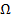), where one Ohm is equal to one Volt per Ampere (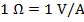).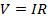V = voltage, also known as potential difference (Volts, V)

I = electric current (Amperes, A)

R = resistance (Ohms,)

Ohm's Law Formula Questions:

1) A current of 2.00 A is flowing through an electric circuit. What is the potential difference across a resistor, with a resistance of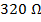?

Answer: The potential difference can be found using the Ohm's Law formula: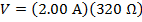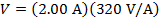V = 640V

The potential difference across the resistor in the circuit is 640 V.

2) A current of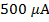is flowing through an electric circuit. What is the potential difference across a resistor, with a resistance of?

Answer: The current in the circuit is given in units of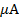, meaning micro-Amperes. One Ampere consists of one million micro-Amperes:. The current is therefore: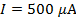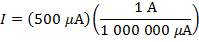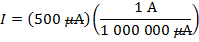The resistance is given in units of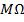, meaning mega-Ohms. One mega-Ohm is equal to one million Ohms: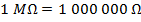. The resistance is therefore: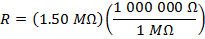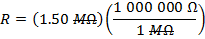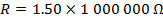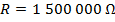Now, the potential difference can be found using the Ohm's Law formula: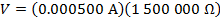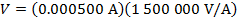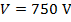The potential difference across the resistor in the circuit is 750 V.